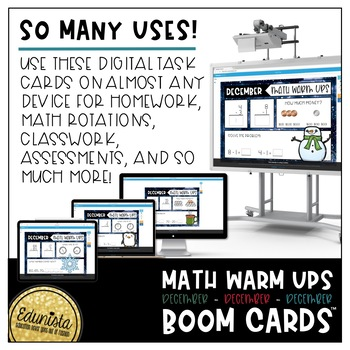# Math Warm Up December Boom Cards™ Digital Task Cards - Differentiated 2 LevelsResource Type
File Type

PDF

(11 MB|46 pages)
Product Rating
Standards
Also included in:
1. Daily Math Warm Ups Boom Cards™ give students the practice they need to maintain basic math skills. These digital task cards target counting, addition, subtraction, measurement, counting coins and telling time. Quick practice - 3 skills targeted each day, designed to be done quickly so that time to
\$45.00
\$22.50
Save \$22.50
• Product Description
• StandardsNEW

Daily Math Warm Ups give students the practice they need to maintain basic math skills. These math warm ups target counting, addition, subtraction, measurement, counting coins and telling time. Quick practice - 3 skills targeted each day, designed to be done quickly so that time to learn new skills is maximized! Differentiated - 2 levels to better meet students’ needs!

We have included 23 cards in each set to allow your students to practice this same set many, many times! Randomly reassign 1 of the 23 cards to your students each day for practice or assessments to gather multiple data points!

Lower Level:

★ single digit addition and subtraction in vertical and horizontal formats

★ counting up and down by increments of one

★ telling time to the half hour accuracy

★ counting groups of same coins

★ measuring length to the inch accuracy

Higher Level:

★ 2-digit addition and subtraction in vertical format, some requiring regrouping

★ 2- and 3- digit addition and subtraction in horizontal format

★ counting up and down by increments of five and ten; also counting down by ones

★ telling time to the five minute accuracy

★ counting groups of mixed value coins

★ measuring length to the half inch accuracy

Math warm ups are designed to help students generalize math skills by:

→ providing calculation problems in both vertical and horizontal formats

→ showing fronts and backs of each type of coin

Math warm ups are designed to:

→ encourage students to find and use mathematical patterns

→ give students the space struggling writers need to respond by maximizing white space

→ capture seasonal fun with graphics while keeping the same overall look in both the lower and higher levels

→ increase student independence with a field of three answer options when telling time to the hour and counting coins in the lower level set

We created Math Warm Ups to give our kiddos quick practice with essential skills in a format that maximized their independence. We hope it works well for your students too!

The Math Warm Ups Single Digit Boom Cards™ cover the following Common Core standards:

CCSS.MATH.CONTENT.1.OA.C.6:

Add and subtract within 20, demonstrating fluency for addition and subtraction within 10.

CCSS.MATH.CONTENT.1.MD.B.3:

Tell and write time in hours and half-hours using analog and digital clocks.

CCSS.MATH.CONTENT.2.MD.A.1:

Measure the length of an object by selecting and using appropriate tools such as rulers, yardsticks, meter sticks, and measuring tapes.

CCSS.MATH.CONTENT.1.NBT.A.1:

Count to 120, starting at any number less than 120.

The Math Warm Ups Double Digit Boom Cards™ cover the following Common Core standards:

CCSS.MATH.CONTENT.2.OA.B.2:

Fluently add and subtract within 20 using mental strategies.

CCSS.MATH.CONTENT.2.MD.C.7:

Tell and write time from analog and digital clocks to the nearest five minutes, using a.m. and p.m.

CCSS.MATH.CONTENT.2.MD.A.1:

Measure the length of an object by selecting and using appropriate tools such as rulers, yardsticks, meter sticks, and measuring tapes.

CCSS.MATH.CONTENT.2.NBT.A.2:

Count within 1000; skip-count by 5s, 10s, and 100s.

Fluently add and subtract within 20 using mental strategies. By end of Grade 2, know from memory all sums of two one-digit numbers.
Add and subtract within 20, demonstrating fluency for addition and subtraction within 10. Use strategies such as counting on; making ten (e.g., 8 + 6 = 8 + 2 + 4 = 10 + 4 = 14); decomposing a number leading to a ten (e.g., 13 - 4 = 13 - 3 - 1 = 10 - 1 = 9); using the relationship between addition and subtraction (e.g., knowing that 8 + 4 = 12, one knows 12 - 8 = 4); and creating equivalent but easier or known sums (e.g., adding 6 + 7 by creating the known equivalent 6 + 6 + 1 = 12 + 1 = 13).
Count within 1000; skip-count by 5s, 10s, and 100s.
Count to 120, starting at any number less than 120. In this range, read and write numerals and represent a number of objects with a written numeral.
Tell and write time from analog and digital clocks to the nearest five minutes, using a.m. and p.m.
Total Pages
46 pages
Included
Teaching Duration
1 month
Report this Resource to TpT
Reported resources will be reviewed by our team. Report this resource to let us know if this resource violates TpT’s content guidelines.\$2.25
List Price:
\$4.50
You Save:
\$2.25